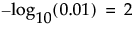The p-value for the significance test corresponding to the FRatio. See Effect Tests in the Fitting Linear Models book for additional details about Effect Tests.
The quantity -log10(p-value). This transformation adjusts p-values to provide an appropriate scale for graphing. A value that exceeds 2 is significant at the 0.01 level (because).
The False Discovery Rate p-value calculated using the Benjamini-Hochberg technique. This technique adjusts the p-values to control the false discovery rate for multiple tests. For details about the FDR correction, see Benjamini and Hochberg, 1995. For details about the false discovery rate, see The False Discovery Rate or Westfall et al. (2011).
The quantity -log10(FDR PValue). This is the best statistic for plotting and assessing significance. Note that small p-values result in high FDR LogWorth values.
The rank of the FDR LogWorth expressed as a fraction of the number of tests. If the number of tests is m, the largest FDR LogWorth value has Rank Fraction 1/m, and the smallest has Rank Fraction 1. Equivalently, the Rank Fraction ranks the p-values in increasing order, as a fraction of the number of tests. The Rank Fraction is used in plotting the PValues and FDR PValues in rank order of decreasing significance.

Help created on 9/19/2017